### Sidebar

Categories

kb:projects:midi_controller:using_midi_controller_with_planetcnc_tng_software

# Using MIDI controller with PlanetCNC TNG software

This project will describe how to use MIDI controller with PlanetCNC TNG software to expand software functionality .

## Introduction

MIDI controllers are usually associated with music production environment, where MIDI controller represents either a keyboard, synthesizer, touch pad etc.. Such devices are usually populated with numerous buttons, keys, pads, sliders, potentiometers and encoders.

The idea is, using a MIDI device as a machine control panel to adjust jogging speed, override values as also setting up work positions values and NC program control…

Device that we will use is AKAI LPD8: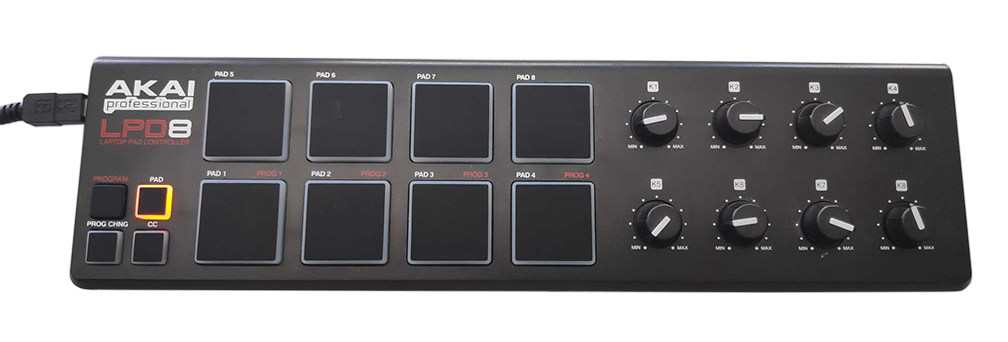This document will cover following topics:

• setting up a MIDI device in PlanetCNC TNG
• identifying MIDI device data
• device button functionality and button data interpretation
• creating expression script file

We recommend reading trough reference documentation of MIDI functions and program commands:
MIDI Functions
Commands

## Setting up a MIDI device in PlanetCNC TNG

First we need to set our MIDI device in PlanetCNC TNG as an input device. This is done in settings under: File/Settings/User Interface/Utilities/Midi/MidiIn.By enabling the Debug option, we can receive immediate feedback from a device in the output window. This is a great way to see if TNG and device communicate and if everything is ok up to this point. It also give us idea what messages are buttons and knobs sending.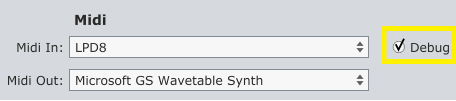We can already observe the data from the device, such as device name, midi channel, type of midi message and message values: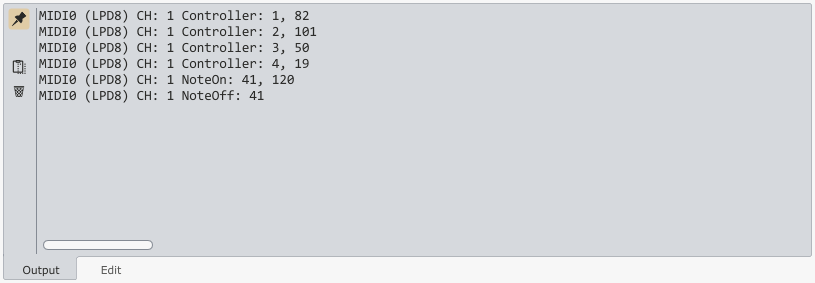But this info is not really helpful besides informational use. That is why we will use #OnMidiIn built-in expression function.

## Identifying MIDI device message data

Let's create an expression file named: Expr_MIDI.txt. Include the code below:

```#OnMidiIn

print("#OnMidiIn: idx=", .arg1, ", name=", .arg2, ", typ=", .arg3, ", ch=", .arg4, ", val1=", .arg5, ", val2=", .arg6);```

#OnMidiIn is a built-in expression function that receives six arguments. These arguments are message data from a midi device, and are already parsed and passed from an external process. Any activity on MIDI device(button/pad pressed, encoder rotation…) will be translated as a printed argument values in the output window.

This is a great diagnostic tool for MIDI messages.

Video below shows printed values:

## Device button functionality and button data interpretation

Image below describes, which functionality I would like to assign to certain pad or potentiometer: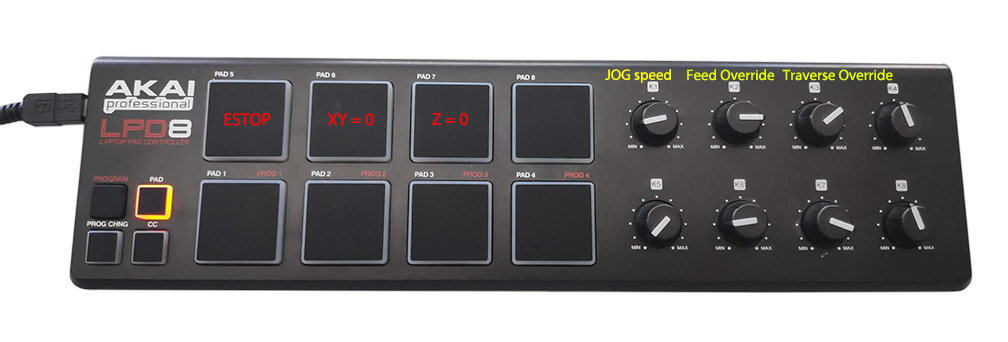### ESTOP button

For Estop I will use PAD5. If I toggle PAD5 and observe the output window, I can see printed text as below: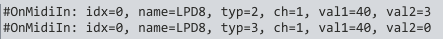typ:
Value 2 is when pad is pressed
Value 3 is when pad is released

val1:
Value 40 is unique for this pad.

This means, that I will evaluate values .arg3(typ) = 2 and .arg5(val1) = 40 for this pad.

### XY = 0 button

For XY = 0 I will use PAD6. If I toggle PAD6 and observe the output window, I can see printed text as below: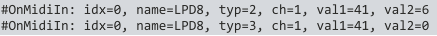typ:
Value 2 is when pad is pressed
Value 3 is when pad is released

val1:
Value 41 is unique for this pad.

This means, that I will evaluate values .arg3(typ) = 2 and .arg5(val1) = 41 for this pad.

### Z = 0 button

For Z=0 I will use PAD7. If I toggle PAD7 and observe the output window, I can see printed text as below: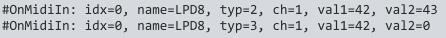typ:
Value 2 is when pad is pressed
Value 3 is when pad is released

val1:
Value 42 is unique for this pad.

This means, that I will evaluate values .arg3(typ) = 2 and .arg5(val1) = 42 for this pad.

For JOG speed adjust I will use K1 encoder. If I rotate K1 and observe the output window, I can see printed text as below: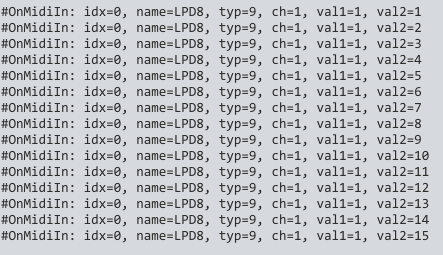typ:
Value is 9, when encoders are rotated

val1:
Value 1 is when K1 encoder is rotated

val2:
Value changes in a range 0-127.

This means, that I will evaluate values: .arg5(val1) = 1 and .arg6(val2) = [0..127] for this encoder.

For Feed override adjust I will use K2 encoder. If I rotate K2 and observe the output window, I can see printed text as below: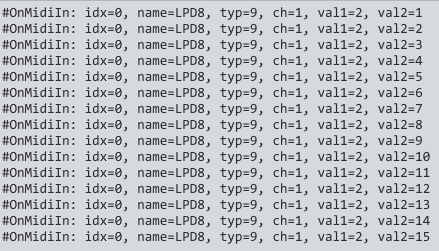typ:
Value is 9, when encoders are rotated

val1:
Value 2 is when K2 encoder is rotated

val2:
Value changes in a range 0-127.

This means, that I will evaluate values: .arg5(val1) = 1 and .arg6(val2) = [0..127] for this encoder.

For Traverse adjust I will use K3 encoder. If I rotate K3 and observe the output window, I can see printed text as below: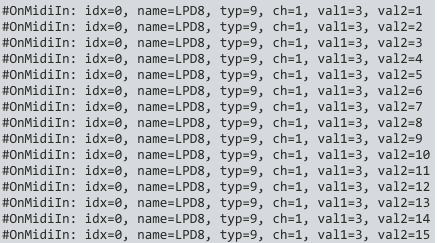typ:
Value is 9, when encoders are rotated

val1:
Value 3 is when K3 encoder is rotated

val2:
Value changes in a range 0-127.

This means, that I will evaluate values: .arg5(val1) = 1 and .arg6(val2) = [0..127] for this encoder.

Table below describes buttons and encoders with their corresponding values, that we will use for further script code:

PAD/Encoder Functionality .arg3(pritned as “typ”) .arg5(val1) .arg6(val2)
K1 encoder JOG speed adjust 9 1 0..127
K2 encoder Feed speed override adjust 9 2 0..127
K3 encoder Traverse speed override adjust 9 3 0..127

## Creating expression script file

### Example 1

Implements PAD5 of MIDI controller as Estop button.

```#OnMidiIn

;according to the table, .arg3 and .arg5 will change if button is pressed. They are saved to private variables .button and .type
.button = .arg5;
.type = .arg3;

;.type should have value 2 and .button should have value 40 when Estop button is pressed. If condition is true, estop is activated
if(.button == 40 && .type == 2,
estop()
);```

### Example 2

Implements PAD6 of MIDI controller as work position XY = 0

```#OnMidiIn

;according to the table, .arg3 and .arg5 will change if button is pressed. They are saved to private variables .button and .type
.button = .arg5;
.type = .arg3;

;.type should have value 2 and .button should have value 41 when XY = 0 button is pressed. If condition is true, work position XY = 0 is set
if(.button == 41 && .type == 2,
cmd("Machine.Work_Position.Axis_To_Zero.XY")
);```

### Example 3

Implements PAD7 of MIDI controller as work position Z = 0

```#OnMidiIn

;according to the table, .arg3 and .arg5 will change if button is pressed. They are saved to private variables .button and .type
.button = .arg5;
.type = .arg3;

;.type should have value 2 and .button should have value 42 when Z = 0 button is pressed. If condition is true, work position Z = 0 is set
if(.button == 42 && .type == 2,
cmd("Machine.Work_Position.Axis_To_Zero.Z")
);```

### Example 4

Implements K1 encoder of MIDI controller as JOG speed adjust encoder

```#OnMidiIn

;.arg5 value is saved to private variable .button
.button = .arg5;

;checks if .button is rotated.
;result of division of .arg6/127 is saved to private variable .value. Value is rounded to 2 decimals in a range 0..1
;.value is multiplied with global parameter _jog_speeddef, and assigned to global parameter _jog_speed that sets current jogging speed
if(.button == 1,
exec(
.value = .arg6/127,
.value = round(value, 2),
_jog_speed = _jog_speeddef * .value
)
);```

### Example 5

Implements K2 and K3 encoder of MIDI controller as Feed override and Traverse override adjust encoders

```#OnInit
debug = 0;

#OnMidiIn

print("#OnMidiIn: idx=", .arg1, ", name=", .arg2, ", typ=", .arg3, ", ch=", .arg4, ", val1=", .arg5, ", val2=", .arg6);

button = .arg5;

if(button == 2,
if(.arg6 <= 64,
exec(
value = .arg6/64,
value = value / _ovrd_speedfeed_stp,
value = round(value),
value = value * _ovrd_speedfeed_stp,
cmd("Machine.Override.Feed.Reset", value),
if(value < _ovrd_speedfeed_min, cmd("Machine.Override.Feed.Reset", _ovrd_speedfeed_min))
),
if(.arg6 > 64,
exec(
value = (.arg6-64)/(63/(_ovrd_speedfeed_max - 1))+1,
value = value / _ovrd_speedfeed_stp,
value = round(value),
value = value * _ovrd_speedfeed_stp,
cmd("Machine.Override.Feed.Reset", value)
)
)
)
);

if(button == 3,
if(.arg6 <= 64,
exec(
value = .arg6/64,
value = value /_ovrd_speedtraverse_stp,
value = round(value),
value = value * _ovrd_speedtraverse_stp,
cmd("Machine.Override.Traverse.Reset", value),
if(value < _ovrd_speedtraverse_min, cmd("Machine.Override.Traverse.Reset", _ovrd_speedtraverse_min))
),
if(.arg6 > 64,
exec(
value = (.arg6-64)/(63/(_ovrd_speedtraverse_max - 1))+1,
value = value / _ovrd_speedtraverse_stp,
value = round(value),
value = value * _ovrd_speedtraverse_stp,
cmd("Machine.Override.Traverse.Reset", value)
)
)
)
);
```